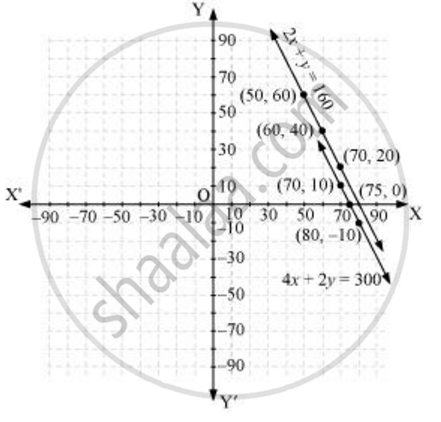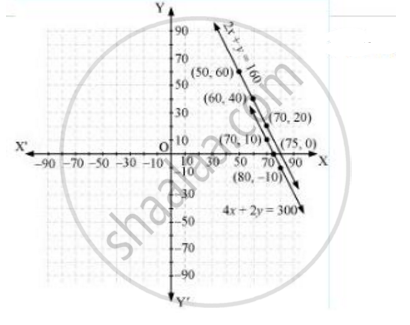# The cost of 2 kg of apples and 1 kg of grapes on a day was found to be Rs 160. After a month, the cost of 4 kg of apples and 2 kg of grapes is Rs 300. Represent the situation algebraically and geometrically. - Mathematics

The cost of 2 kg of apples and 1 kg of grapes on a day was found to be Rs 160. After a month, the cost of 4 kg of apples and 2 kg of grapes is Rs 300. Represent the situation algebraically and geometrically.

#### Solution 1

Let the cost of 1 kg of apples be Rs x.

And, cost of 1 kg of grapes = Rs y

According to the question, the algebraic representation is

2x + y = 160

4x + 2y = 300

For 2x + y = 160

y = 160 - 2x

The solution table is

 x 50 60 70 y 60 40 20

For 4x + 2y = 300,

y = (300 - 4x)/2

The solution table is

 x 70 80 75 y 10 −10 0

The graphical representation is as follows#### Solution 2

Cost per kg of apple = Rs x

Cost per kg of grapes = Rs y

Algerbraically 2x + y = 160 ...........(1)

4x + 2y = 300 or 2x + y = 150 ..........(2)

From (1) y = 160 - 2x

 x 50 60 Y = 160 - 2x 60 40

From (2), y = 150 - 2x

 x 50 60 Y = 150 - 2x 50 30

The graphical representation is as followConcept: Pair of Linear Equations in Two Variables
Is there an error in this question or solution?

#### APPEARS IN

RD Sharma Class 10 Maths
Chapter 3 Pair of Linear Equations in Two Variables
Exercise 3.1 | Q 7 | Page 12
NCERT Class 10 Maths
Chapter 3 Pair of Linear Equations in Two Variables
Exercise 3.1 | Q 2 | Page 44